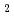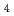A new technique was developed to estimate compositions of Bunsen reaction solution in liquid-liquid phase separation which was targeted on the two solutions in phase equilibrium. In this technique, just two densities measured in the two solution are employed to estimate compositions of two solutions consisting of HI, HSO, I, HO. The fundamental equations underlying this estimation is relations between densities and compositions. To formulate the relations, densities of simulated Bunsen reaction solutions were measured and regression expressions were formulated. Moreover, relational equations for both phases were set up from measured values by the regression procedure. Through estimations of both compositions from both densities, the calculated values are approximately consistent with titrated values. Because of easily and simply execution, this technique is useful for practical use, especially for plant operation and equipment design.